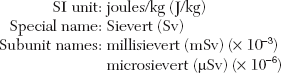# Radiation dose, dosimetry and dose limitation

Several different terms and units have been used in dosimetry over the years. The use of the word dose in multiple different descriptors has made this subject even more confusing. However, it is essential that these terms and units are understood to appreciate what is meant by radiation dose and to allow meaningful comparisons between different investigations to be made. In addition to explaining the various units and how they are measured/calculated, this chapter also covers the concept of clinical dose limits and dose limitation, as well as summarizing the estimated doses from various sources of ionizing radiation and the magnitude of radiation doses from common dental and medical clinical investigations.

The more important terms in dosimetry include:

## Radiation-absorbed dose (D)

This is a measure of the amount of energy absorbed from the radiation beam per unit mass of tissue and can be measured using a dosimeter.## Equivalent dose (HT)

This is a measure that allows the different radio-biological effectiveness (RBE) of different types of radiation to be taken into account.

For example, alpha particles penetrate only a few millimetres in tissue, lose all their energy and are totally absorbed, whereas X-rays penetrate much further, lose some of their energy and are only partially absorbed. The biological effect of a particular radiation-absorbed dose of alpha particles would be considerably more severe than a similar radiation-absorbed dose of X-rays.

By introducing a numerical value known as the radiation weighting factor (WR), which represents the biological effects of different radiations on different tissues, the unit of equivalent dose (HT) in a particular tissue provides a common unit allowing comparisons to be made between one type of radiation and another, for example:

 X-rays, gamma rays and beta particles WR = 1 Fast neutrons (10 keV–100 keV) and protons WR = 10 Alpha particles WR = 20

Equivalent dose (HT) = radiation-absorbed dose (D) × radiation weighting factor (WR) in a particular tissue(For X-rays, the radiation weighting factor (WR) = 1, therefore the equivalent dose (HT) in a particular tissue, measured in Sieverts, is equal to the radiation-absorbed dose (D), measured in Grays.)

Jan 12, 2015 | Posted by in Oral and Maxillofacial Radiology | Comments Off on 4: Radiation dose, dosimetry and dose limitation
Premium Wordpress Themes by UFO Themes